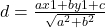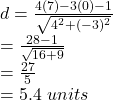## On a coordinate plane, parallelogram R S T U is shown. Point R is at (1, 1), point S is at (7, 0), point T is at (10, 4), and point U is at

Question

On a coordinate plane, parallelogram R S T U is shown. Point R is at (1, 1), point S is at (7, 0), point T is at (10, 4), and point U is at (4, 5).
If line segment RU is considered the base of parallelogram RSTU, what is the corresponding height of the parallelogram?

4.5 units
5.4 units
9.0 units
10.8 units

in progress 0
5 months 2021-08-24T23:31:29+00:00 2 Answers 8 views 0

B,5.4 units.

Step-by-step explanation:

Parallelogram RSTU

R(1,1),S(7,0),T(10,4),U(4,5)

slope of RU m=(5-1)/(4-1)=4/3

slope of RS=(0-1)/(7-1)=-1/6=-1/6

slope of ST=(4-0)/(10-7)=4/3

slope of  TU=(5-4)/(4-10)=1/(-6)=-1/6

RU||ST

eq. of line RU is

y-y1=m(x-x1)

y-1=4/3(x-1)

3y-3=4x-4

4x-3y-4+3=0

4x-3y-1=0

perpendicular distance of a point (x1,y1) from line ax+by+c=0 isperpendicular distance of (7,0) from 4x-3y-1=0